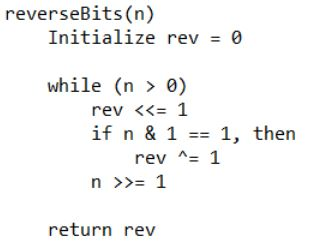# Reverse actual bits of the given number

Given a non-negative integer n. The problem is to reverse the bits of n and print the number obtained after reversing the bits. Note that the actual binary representation of the number is being considered for reversing the bits, no leading 0’s are being considered.

Examples :

```Input : 11
Output : 13
(11)10 = (1011)2.
After reversing the bits we get:
(1101)2 = (13)10.

Input : 10
Output : 5
(10)10 = (1010)2.
After reversing the bits we get:
(0101)2 = (101)2
= (5)10.
```

## Recommended: Please try your approach on {IDE} first, before moving on to the solution.

In this approach, one by one bits in binary representation of n are being obtained with the help of bitwise right shift operation and they are being accumulated in rev with the help of bitwise left shift operation.
Algorithm:## C++

 `// C++ implementation to reverse bits of a number ` `#include ` ` `  `using` `namespace` `std; ` ` `  `// function to reverse bits of a number ` `unsigned ``int` `reverseBits(unsigned ``int` `n) ` `{ ` `    ``unsigned ``int` `rev = 0; ` `     `  `    ``// traversing bits of 'n' from the right ` `    ``while` `(n > 0) ` `    ``{ ` `        ``// bitwise left shift  ` `        ``// 'rev' by 1 ` `        ``rev <<= 1; ` `         `  `        ``// if current bit is '1' ` `        ``if` `(n & 1 == 1) ` `            ``rev ^= 1; ` `         `  `        ``// bitwise right shift  ` `        ``// 'n' by 1 ` `        ``n >>= 1; ` `             `  `    ``} ` `     `  `    ``// required number ` `    ``return` `rev; ` `} ` ` `  `// Driver program to test above ` `int` `main() ` `{ ` `    ``unsigned ``int` `n = 11; ` `    ``cout << reverseBits(n); ` `    ``return` `0; ` `} `

## Java

 `// Java implementation to  ` `// reverse bits of a number ` `class` `GFG  ` `{ ` `    ``// function to reverse bits of a number ` `    ``public` `static` `int` `reverseBits(``int` `n) ` `    ``{ ` `        ``int` `rev = ``0``; ` ` `  `        ``// traversing bits of 'n'  ` `        ``// from the right ` `        ``while` `(n > ``0``)  ` `        ``{ ` `            ``// bitwise left shift  ` `            ``// 'rev' by 1 ` `            ``rev <<= ``1``; ` ` `  `            ``// if current bit is '1' ` `            ``if` `((``int``)(n & ``1``) == ``1``) ` `                ``rev ^= ``1``; ` ` `  `            ``// bitwise right shift  ` `            ``//'n' by 1 ` `            ``n >>= ``1``; ` `        ``} ` `        ``// required number ` `        ``return` `rev; ` `    ``} ` ` `  `    ``// Driver code  ` `    ``public` `static` `void` `main(String[] args) ` `    ``{ ` `        ``int` `n = ``11``; ` `        ``System.out.println(reverseBits(n)); ` `    ``} ` `} ` ` `  `// This code is contributed  ` `// by  prerna saini. `

## Python 3

 `# Python 3 implementation to  ` `# reverse bits of a number ` ` `  ` `  `# function to reverse  ` `# bits of a number ` `def` `reverseBits(n) : ` `     `  `    ``rev ``=` `0` `     `  `    ``# traversing bits of 'n' from the right ` `    ``while` `(n > ``0``) : ` `         `  `        ``# bitwise left shift 'rev' by 1 ` `        ``rev ``=` `rev << ``1` `         `  `        ``# if current bit is '1' ` `        ``if` `(n & ``1` `=``=` `1``) : ` `            ``rev ``=` `rev ^ ``1` `         `  `        ``# bitwise right shift 'n' by 1 ` `        ``n ``=` `n >> ``1` `         `  `     `  `    ``# required number ` `    ``return` `rev ` `     `  `# Driver code ` `n ``=` `11` `print``(reverseBits(n)) ` ` `  ` `  `# This code is contributed  ` `# by Nikita Tiwari. `

## C#

 `// C# implementation to  ` `// reverse bits of a number ` `using` `System; ` `class` `GFG  ` `{ ` `    ``// function to reverse bits of a number ` `    ``public` `static` `int` `reverseBits(``int` `n) ` `    ``{ ` `        ``int` `rev = 0; ` ` `  `        ``// traversing bits of 'n'  ` `        ``// from the right ` `        ``while` `(n > 0)  ` `        ``{ ` `            ``// bitwise left shift  ` `            ``// 'rev' by 1 ` `            ``rev <<= 1; ` ` `  `            ``// if current bit is '1' ` `            ``if` `((``int``)(n & 1) == 1) ` `                ``rev ^= 1; ` ` `  `            ``// bitwise right shift  ` `            ``//'n' by 1 ` `            ``n >>= 1; ` `        ``} ` `        ``// required number ` `        ``return` `rev; ` `    ``} ` ` `  `    ``// Driver code  ` `    ``public` `static` `void` `Main() ` `    ``{ ` `        ``int` `n = 11; ` `        ``Console.WriteLine(reverseBits(n)); ` `    ``} ` `} ` ` `  `// This code is contributed  ` `// by vt_m. `

## PHP

 ` 0) ` `    ``{ ` `        ``// bitwise left shift  ` `        ``// 'rev' by 1 ` `        ``\$rev` `<<= 1; ` `         `  `        ``// if current bit is '1' ` `        ``if` `(``\$n` `& 1 == 1) ` `            ``\$rev` `^= 1; ` `         `  `        ``// bitwise right shift  ` `        ``// 'n' by 1 ` `        ``\$n` `>>= 1; ` `             `  `    ``} ` `     `  `    ``// required number ` `    ``return` `\$rev``; ` `} ` ` `  `// Driver code ` `\$n` `= 11; ` `echo` `reverseBits(``\$n``); ` ` `  `// This code is contributed by mits  ` `?> `

Output :

```13
```

Time Complexity: O(num), where num is the number of bits in the binary representation of n.

My Personal Notes arrow_drop_upCheck out this Author's contributed articles.

If you like GeeksforGeeks and would like to contribute, you can also write an article using contribute.geeksforgeeks.org or mail your article to contribute@geeksforgeeks.org. See your article appearing on the GeeksforGeeks main page and help other Geeks.

Please Improve this article if you find anything incorrect by clicking on the "Improve Article" button below.

Improved By : Mithun Kumar

Article Tags :
Practice Tags :

5

Please write to us at contribute@geeksforgeeks.org to report any issue with the above content.